Courses

# NCERT Exemplar - Force and Laws of Motion Class 9 Notes | EduRev

## Class 9 : NCERT Exemplar - Force and Laws of Motion Class 9 Notes | EduRev

The document NCERT Exemplar - Force and Laws of Motion Class 9 Notes | EduRev is a part of the Class 9 Course Science Class 9.
All you need of Class 9 at this link: Class 9

Q.1. Two balls of the same size but of different materials, rubber and iron are kept on the smooth floor of a moving train. The brakes are applied suddenly to stop the train. Will the balls start rolling? If so, in which direction? Will they move with the same speed? Give reasons for your answer.
Ans: Yes, both the balls will start rolling in forward direction. As iron ball has greater inertia, so it will roll with less speed.

Q.2. Velocity versus time graph of a ball of mass 50 g rolling on a concrete floor is shown in the figure. Calculate the acceleration and frictional force of the floor on the ball.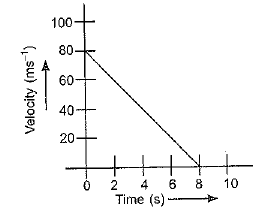Ans: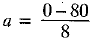= - 10 m/s2 i.e. in opposite direction to motion.
F = ma =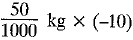= - 0.5 N i.e. in opposite direction to motion.

Q.3. Two identical bullets are fired one by a light rifle and another by a heavy rifle with the same force. Which rifle will hurt the shoulder more and why?
Ans: The light rifle will hurt the shoulder more as greater acceleration will be produced in it.

Q.4. A horse continues to apply a force in order to move a cart with a constant speed. Explain why?
Ans: The horse has to continuously apply the force in order to balance the frictional force acting between cart-wheels and road.

Q.5. Suppose a ball of mass m is thrown vertically upward with an initial speed v. Its speed decreases continuously till it becomes zero. Thereafter, the,ball begins . to fall-downward and attains the speed v again before striking the ground. It implies that the magnitude of initial and final momenta of the ball are same. Yet, it is not an example of conservation of momentum. Explain why?
Ans: In this case, the change in momentum occurs due to unbalanced force of gravity. However, the law of conservation of momentum requires a situation in which no external force acts.

Q.6. A truck of mass M is moved under a force F. If the truck is then loaded with an object equal to the mass of the truck and the driving force is halved, then how does the acceleration change?
Ans: F = ma
When M changes to 2M and F changes to F/2, then acceleration a =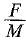changes to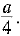Q.7. Two friends on roller-skates are standing 5 m apart facing each other. One of them throws ball of 2 kg towards the other, who catches it. How will this activity affect the position of the two? Explain your answer.
Ans: The one who throws the ball and the one who catches it, both will be pushed backwards due to the reaction force of fall.

Q.8. Water sprinkler used for grass lawns begins to rotate as soon as the water is supplied. Explain the principle on which it works.

Ans: Reaction force exerted by water that comes out at different angles from the sprinkler, causes it to rotate.

Q.9. Using second law of motion, derive the relation between force and acceleration. A bullet of 10 g strikes a sand-bag at a speed of 103 ms1 and gets embedded after travelling 5 cm. Calculate
(i) the resistive force exerted by the sand on the bullet.
(ii) the time taken by the bullet to come to rest.
Ans:
Let a body of mass m moving with velocity u, is acted upon by force F for time t which changes its velocity to v.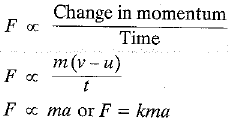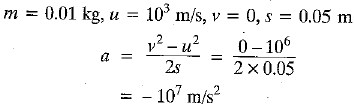(i) F = ma = 0.01 x 107 = 109 N
(ii)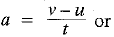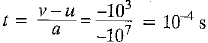Q.10. Derive the unit of force using the second law of motion. A force of 5 N produces an acceleration of 8ms-2 on a mass  mand an acceleration of 24 ms-2 on a mass m2. What acceleration would the same force provide if both the masses are tied together?
Ans: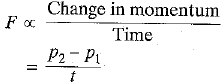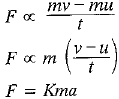F = Kma
∵ K = 1
∴ K = ma
1 N = 1 kg m/s2
F = 5 N, a1 =8 m/s2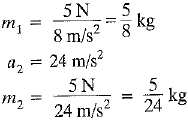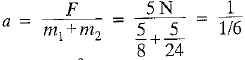= 6 m/s2

Q.11. What is momentum? Write its SI unit. Interpret force in terms of momentum. Represent th following graphically:
(a) momentum versus velocity when mass is fixed.
(b) momentum versus mass when velocity is constant.

Ans: The product of mass and velocity of an object is momentum. Its SI unit is Kg m/s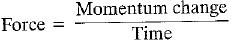(According to second law of motion)
(a)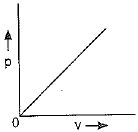(b)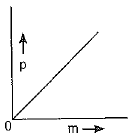Offer running on EduRev: Apply code STAYHOME200 to get INR 200 off on our premium plan EduRev Infinity!

## Science Class 9

143 videos|224 docs|135 tests

,

,

,

,

,

,

,

,

,

,

,

,

,

,

,

,

,

,

,

,

,

;